# Quiz 9: Flexible Budgeting and Variance Analysis

Variance refers to the difference between actual expenses and the estimated or budgeted expenses. Such variances are investigated in order to identify the cause of variance and further actions are taken by the managers to control the causes of variances. Managers generally put an effort towards the understanding of variances better and anticipate the causes which can be occurred in future. Manager compares the line by line of actual and budgeted expenses for each department or cost center of the heath care organization. Difference between the actual and budgeted expenses will always occur. If entire variances are investigated by the managers then a great deal of time will be wasted. Thus manager should investigate only that variance which needs more attentions. Thus, it can be concluded that given statement is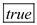Price variance: Price variance is the difference between actual price and estimated price. It can be calculated as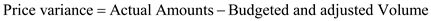Quantity Variance: Quantity variance is difference between the budgeted quantity used and actual quantity used. It is calculated as: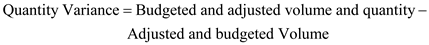Volume Variance: Volume is the difference between adjusted budget and original budget.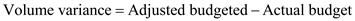It is required to calculate following variance by using given data: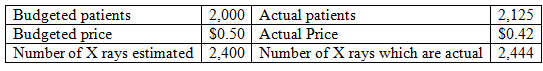a. Provided Original budget is \$1,200 and Actual Expenditures is \$1,026: Total Variance:Thus, Total variance is unfavorable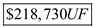b. Volume variance: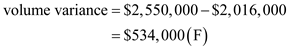Thus, Volume variance is favorable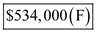c. Quantity variance: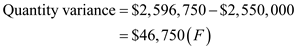Thus, Quantity Variance is favorable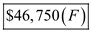d. Price variance: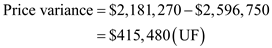Thus price variance is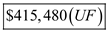Variance of a variable is defined as the difference between the actual, observed values of the variable from the expected or the predicted values of the variable. Volume variance can be calculated by the following formula: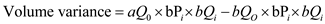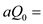Actual quantity of output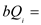Budgeted quantity of input per unit of output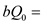Budgeted quantity of output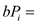Budgeted price of input per unit of output Now, substituting the given values in the above formula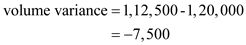Since the actual volume exceeds the budgeted quantity, hence volume variance is calculated to be negative as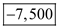units. Quantity variance can be calculated by the following formula: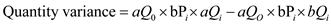Where,Actual quantity of outputBudgeted price of input per unit of output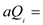Actual quantity of input per unit of outputBudgeted quantity of input per unit of output Now, substituting the given values in the above formula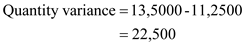Since, the actual quantity at budgeted price exceeds the budgeted quantity, quantity variance is positive and evaluated as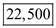Price variance can be calculated by the following formula: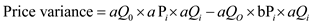Where,Actual quantity of outputBudgeted price of input per unit of outputActual quantity of input per unit of output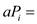Actual price of input per unit of output Now, substituting the given values in the above formula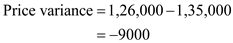Since, the actual quantity at actual price is less than actual quantity at budgeted price, price variance is negative to be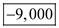Total variance is the sum of quantity variance, volume variance and price variance.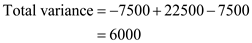Volume variance is negative indicating lesser number of patients against the expected number which is an unfavorable outcome for revenues. Positive Quantity variable is unfavorable because the amount of labor per patient has increased. Price variance is negative indicating a fall in pay rate per labor which is beneficial for revenues.